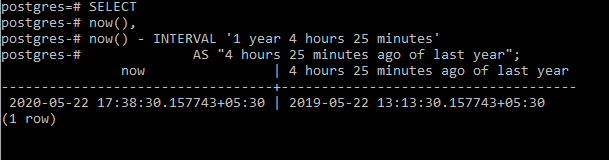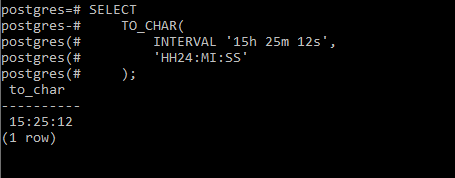Open In App

# PostgreSQL – Interval Data Type

In PostgreSQL the interval data type is used to store and manipulate a time period. It holds 16 bytes of space and ranging from -178, 000, 000 years to 178, 000, 000 years. It also has additional attribute called “precision (denoted by p)” that can be used to set the level of precision in the query results.

```Syntax:interval [ Data_fields ] [ (p) ]

Where,
Data_fields: Time period
p: precision```

PostgreSQL stores the interval type value in the integer form of days and months whereas for values in seconds, it can be fractions.
Now let’s jump into a few examples for demonstration.
Example 1:
In this example we will query to know the time of 4 hours 25 minutes ago at the current time of last year using the below commands:

```SELECT
now(),
now() - INTERVAL '1 year 4 hours 25 minutes'
AS "4 hours 25 minutes ago of last year";```

Output:Example 2:
In this example we will convert an interval value ta string format using the TO_CHAR() function.The TO_CHAR() function takes the first argument as an interval value, the second one as the format, and returns a string that represents the interval in the specified format.

```SELECT
TO_CHAR(
INTERVAL '15h 25m 12s',
'HH24:MI:SS'
);```

Output: